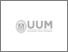UUM Electronic Theses and Dissertation
UUM ETD | Universiti Utara Malaysian Electronic Theses and Dissertation
FAQs | Feedback | Search Tips | Sitemap

# One step hybrid block methods with generalised off-step points for solving directly higher order ordinary differential equations

Abdelrahim, Ra'ft Abdelmajid Moh'd (2016) One step hybrid block methods with generalised off-step points for solving directly higher order ordinary differential equations. PhD. thesis, Universiti Utara Malaysia.Preview
Text
s94643_01.pdfPreview
Text
s94643_02.pdf

## Abstract

Real life problems particularly in sciences and engineering can be expressed in differential
equations in order to analyse and understand the physical phenomena. These differential equations involve rates of change of one or more independent variables. Initial value problems of higher order ordinary differential equations are conventionally
solved by first converting them into their equivalent systems of first order ordinary
differential equations. Appropriate existing numerical methods will then be employed to solve the resulting equations. However, this approach will enlarge the number of equations. Consequently, the computational complexity will increase and thus may jeopardise the accuracy of the solution. In order to overcome these setbacks, direct methods were employed. Nevertheless, most of these methods approximate numerical solutions at one point at a time. Therefore, block methods were then introduced with the aim of approximating numerical solutions at many points simultaneously. Subsequently,
hybrid block methods were introduced to overcome the zero-stability barrier occurred in the block methods. However, the existing one step hybrid block methods only focus on the specific off-step point(s). Hence, this study proposed new one step
hybrid block methods with generalised off-step point(s) for solving higher order ordinary
differential equations. In developing these methods, a power series was used as an approximate solution to the problems of ordinary differential equations of order g. The power series was interpolated at g points while its highest derivative was collocated at all points in the selected interval. The properties of the new methods such as order, error constant, zero-stability, consistency, convergence and region of absolute stability were also investigated. Several initial value problems of higher order ordinary
differential equations were then solved using the new developed methods. The numerical results revealed that the new methods produced more accurate solutions than the existing methods when solving the same problems. Hence, the new methods are viable alternatives for solving initial value problems of higher order ordinary differential
equations directly.

Item Type: Thesis (PhD.) Omar, Zurni 6177 Interpolation, collocation, one step hybrid block method, direct solution, higher order initial value problems, generalised off-step point(s). Q Science > QA Mathematics > QA273-280 Probabilities. Mathematical statisticsQ Science > QA Mathematics > QA299.6-433 Analysis Awang Had Salleh Graduate School of Arts & Sciences 13 Apr 2017 13:09 04 Apr 2021 07:30 Awang Had Salleh Graduate School of Arts and Sciences Omar, Zurni https://etd.uum.edu.my/id/eprint/6177